# Thermo-Texter

Takes the difference between two temperature sensors and texts the results to your phone.

BeginnerFull instructions provided1 hour744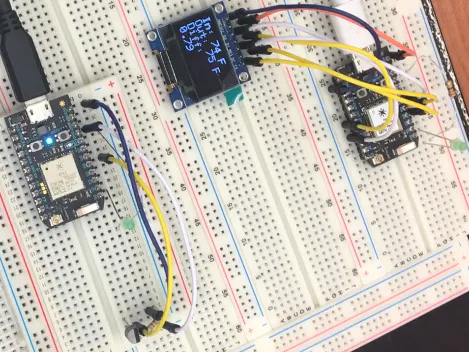## Things used in this project

### Hardware components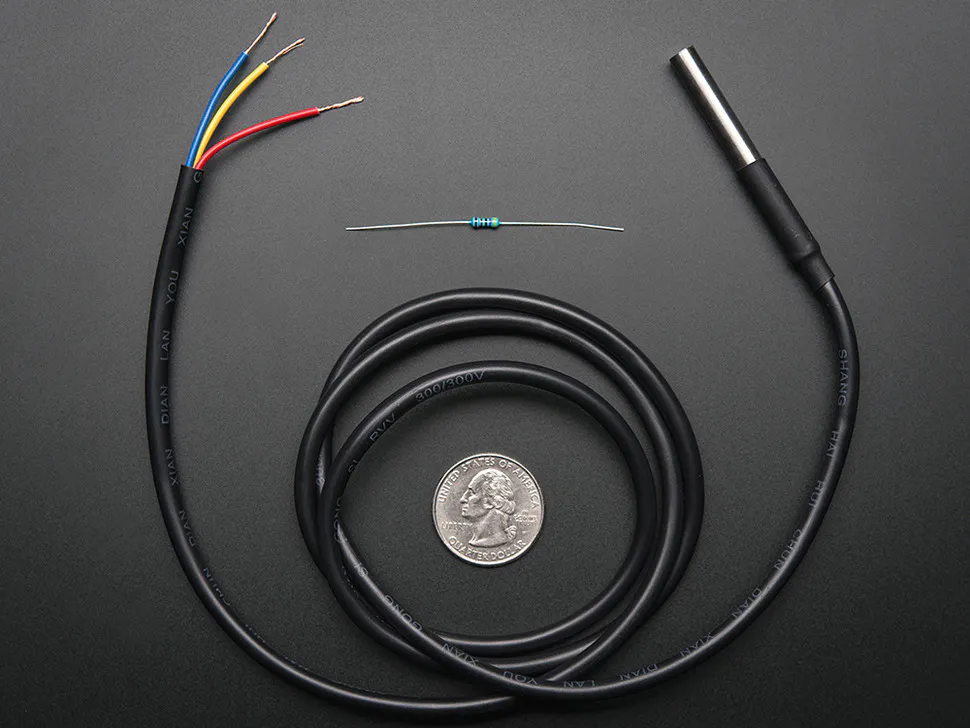Adafruit Waterproof DS18B20 Digital temperature sensor
×1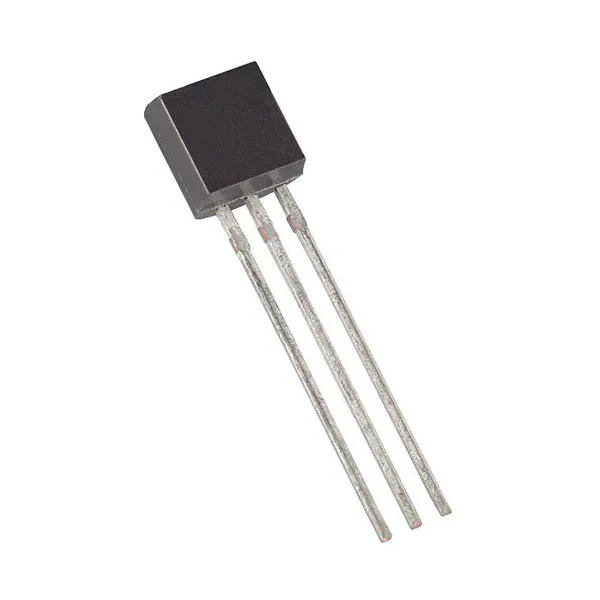Maxim Integrated DS18B20 Programmable Resolution 1-Wire Digital Thermometer
×1LED (generic)
×3
 Arduino SSD1306 OLED
×1Jumper wires (generic)
×7Particle Photon
×3

### Software apps and online services

 IFTT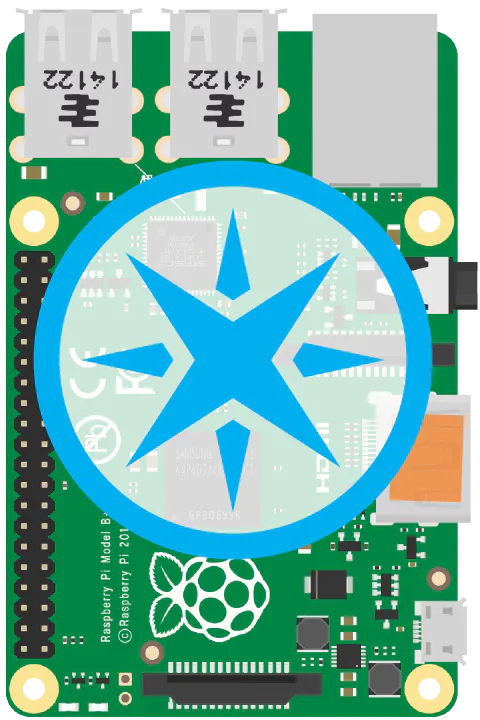Particle Pi

## Schematics

### Primary Photon

Utilizes a 220 Ohm Resistor. Circuit diagram for primary photon that receives and sends texts.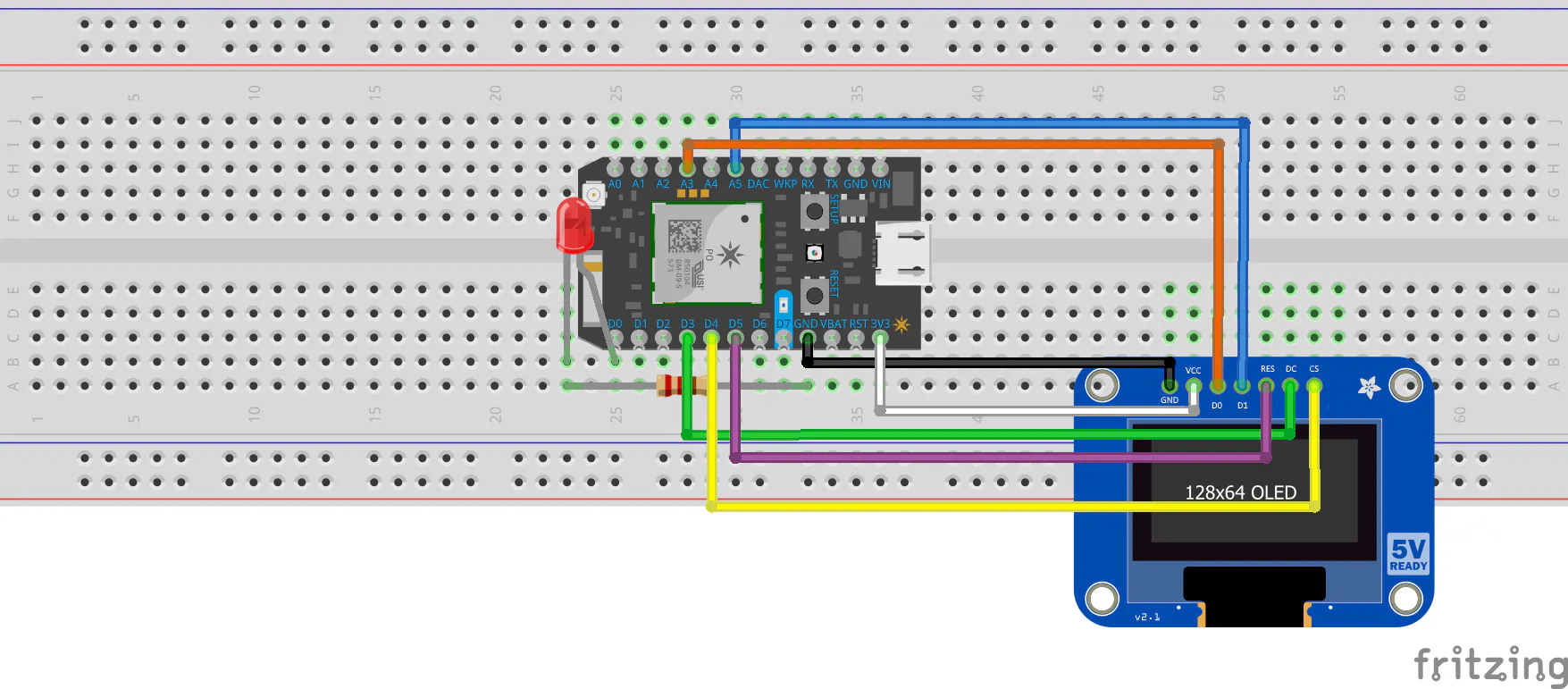### Outside Temperature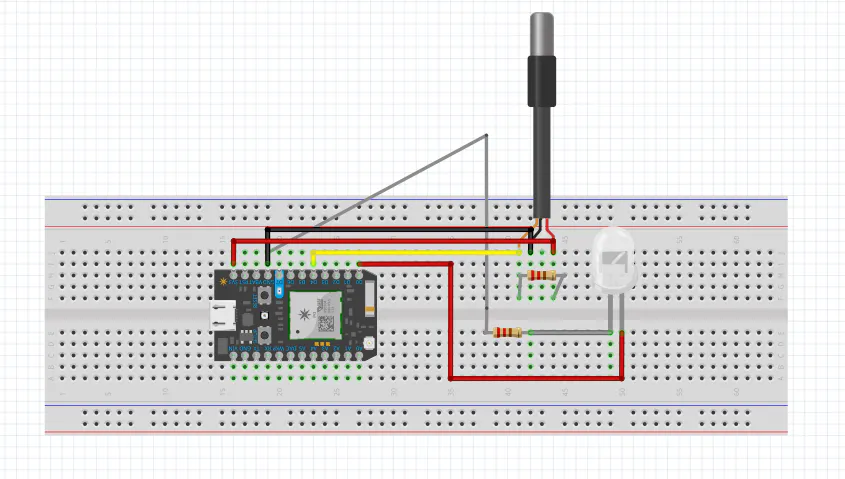### Inside Temperature Photon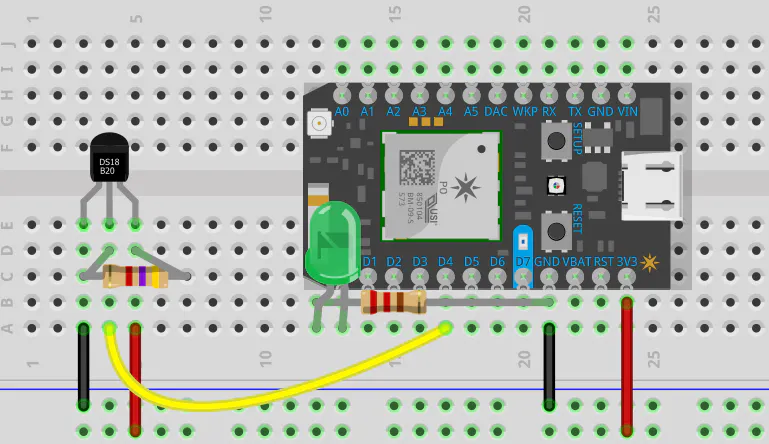## Code

### Primary Photon

C/C++
Receives temperature data from two photons and displays through an OLED screen. Additionally if the user requests the temperature data through text message, the photon will return a text message with the temperature.
```// This #include statement was automatically added by the Particle IDE.

float T1;
float T2;
float difference;

int led1 = D0;

#define OLED_DC     D3
#define OLED_CS     D4
#define OLED_RESET  D5

void setup() {
Particle.subscribe("Naas_temperature", FirstTemp);
Particle.subscribe("0042_temperature", SecondTemp);

Particle.subscribe("TempReturn0595", TempReturn);

pinMode(led1, OUTPUT);

display.begin(SSD1306_SWITCHCAPVCC);

display.setTextSize(2); //Adjust text size here to fit on screen
display.setTextColor(WHITE);
display.setTextWrap(false);
}

void FirstTemp(const char *event, const char *data1) {
T1 = atof(data1);
Serial.print(T1);
}

void SecondTemp(const char *event, const char *data2) {
T2 = atof(data2);
Serial.print(T2);
}

void TempReturn (const char *event, const char *data3) {
String printTemp;

if (strcmp(data3, "True") == 0) {

printTemp = ("Inside temperature: " + String(int(T1)) + "; Outside temperature: " + String(int(T2)) + "; Their exact difference is: " + String(difference)); //Can edit text here to change what is sent to phone
Particle.publish("TempCollect0595", printTemp);

digitalWrite(led1, HIGH);
delay(30000); //Can adjust value to change length of time LED lights (ms)
digitalWrite(led1, LOW);
}
}

void loop() {
if (Particle.connected() == false) {
Particle.connect();
}

Particle.publish("Temp1", String(T1));
Particle.publish("Temp2", String(T2));
difference = T2 - T1;

display.clearDisplay();
display.setCursor(0,0);
display.print("In: ");
display.print(int(T1));
display.println(" F");
display.print("Out: ");
display.print(int(T2));
display.println(" F");
display.println("Diff: ");
display.print(difference);
display.display();
}
```

### Outside Temperature Code

C/C++
Code collects and publishes temperature data as events on the cloud, it also looks for events generated by IFTT temperature requests. IFTT event will trigger an LED light connected to photon, alerting user that the IFTT event has been published.
```// This #include statement was automatically added by the Particle IDE.
#include <OneWire.h>

OneWire ds = OneWire(D4);  // 1-wire signal on pin D4

unsigned long lastUpdate = 0;

float lastTemp;
int led1 = D0;
int led2 = D7;

void setup() {
Serial.begin(9600);
// Set up 'power' pins, comment out if not used!
pinMode(D3, OUTPUT);
pinMode(D5, OUTPUT);
digitalWrite(D3, LOW);
digitalWrite(D5, HIGH);

pinMode(led1, OUTPUT);
pinMode(led2, OUTPUT);
}

void Blinker(const char *event, const char *data) {
if (strcmp(data,"True")==0) {

// To blink the LED, first we'll turn it on...
digitalWrite(led1, HIGH);
digitalWrite(led2, HIGH);

// We'll leave it on for 1 second...
delay(1000);

// Then we'll turn it off...
digitalWrite(led1, LOW);
digitalWrite(led2, LOW);

// Wait 1 second...
delay(1000);

// And repeat!
}
}

// up to here, it is the same as the address acanner
// we need a few more variables for this example

void loop(void) {
byte i;
byte present = 0;
byte type_s;
byte data;
float celsius, fahrenheit;

Serial.println();
ds.reset_search();
delay(250);
return;
}

// The order is changed a bit in this example
// first the returned address is printed

Serial.print("ROM =");
for( i = 0; i < 8; i++) {
Serial.write(' ');
}

// second the CRC is checked, on fail,

Serial.println("CRC is not valid!");
return;
}
Serial.println();

// we have a good address at this point
// what kind of chip do we have?
// we will set a type_s value for known types or just return

// the first ROM byte indicates which chip
case 0x10:
Serial.println("  Chip = DS1820/DS18S20");
type_s = 1;
break;
case 0x28:
Serial.println("  Chip = DS18B20");
type_s = 0;
break;
case 0x22:
Serial.println("  Chip = DS1822");
type_s = 0;
break;
case 0x26:
Serial.println("  Chip = DS2438");
type_s = 2;
break;
default:
Serial.println("Unknown device type.");
return;
}

// this device has temp so let's read it

ds.reset();               // first clear the 1-wire bus
ds.select(addr);          // now select the device we just found
// ds.write(0x44, 1);     // tell it to start a conversion, with parasite power on at the end
ds.write(0x44, 0);        // or start conversion in powered mode (bus finishes low)

// just wait a second while the conversion takes place
// different chips have different conversion times, check the specs, 1 sec is worse case + 250ms
// you could also communicate with other devices if you like but you would need

delay(1000);     // maybe 750ms is enough, maybe not, wait 1 sec for conversion

// we might do a ds.depower() (parasite) here, but the reset will take care of it.

// first make sure current values are in the scratch pad

present = ds.reset();
ds.write(0xB8,0);         // Recall Memory 0
ds.write(0x00,0);         // Recall Memory 0

present = ds.reset();
if (type_s == 2) {
ds.write(0x00,0);       // The DS2438 needs a page# to read
}

// transfer and print the values

Serial.print("  Data = ");
Serial.print(present, HEX);
Serial.print(" ");
for ( i = 0; i < 9; i++) {           // we need 9 bytes
Serial.print(data[i], HEX);
Serial.print(" ");
}
Serial.print(" CRC=");
Serial.print(OneWire::crc8(data, 8), HEX);
Serial.println();

// Convert the data to actual temperature
// because the result is a 16 bit signed integer, it should
// be stored to an "int16_t" type, which is always 16 bits
// even when compiled on a 32 bit processor.
int16_t raw = (data << 8) | data;
if (type_s == 2) raw = (data << 8) | data;
byte cfg = (data & 0x60);

switch (type_s) {
case 1:
raw = raw << 3; // 9 bit resolution default
if (data == 0x10) {
// "count remain" gives full 12 bit resolution
raw = (raw & 0xFFF0) + 12 - data;
}
celsius = (float)raw * 0.0625;
break;
case 0:
// at lower res, the low bits are undefined, so let's zero them
if (cfg == 0x00) raw = raw & ~7;  // 9 bit resolution, 93.75 ms
if (cfg == 0x20) raw = raw & ~3; // 10 bit res, 187.5 ms
if (cfg == 0x40) raw = raw & ~1; // 11 bit res, 375 ms
// default is 12 bit resolution, 750 ms conversion time
celsius = (float)raw * 0.0625;
break;

case 2:
data = (data >> 3) & 0x1f;
if (data > 127) {
celsius = (float)data - ((float)data * .03125);
}else{
celsius = (float)data + ((float)data * .03125);
}
}

// remove random errors
if((((celsius <= 0 && celsius > -1) && lastTemp > 5)) || celsius > 50) {
celsius = lastTemp;
}

fahrenheit = celsius * 1.8 + 32.0;
lastTemp = celsius;
Serial.print("  Temperature = ");
Serial.print(celsius);
Serial.print(" Celsius, ");
Serial.print(fahrenheit);
Serial.println(" Fahrenheit");

// now that we have the readings, we can publish them to the cloud
String temperature = String(fahrenheit); // store temp in "temperature" string
Particle.publish("0042_temperature", temperature, PUBLIC); // publish to cloud
delay(10000); // 60 second delay
}
```

### Inside Temperature Code

C/C++
Measures temperature inside and publishes it to the primary photon; also looks for confirmation of text message and lights an LED.
```// This #include statement was automatically added by the Particle IDE.
#include <OneWire.h>

OneWire ds = OneWire(D4);  // 1-wire signal on pin D4

unsigned long lastUpdate = 0;

float lastTemp;
int led1 = D0;
int led2 = D7;

void setup() {
Serial.begin(9600);
// Set up 'power' pins, comment out if not used!
pinMode(D3, OUTPUT);
pinMode(D5, OUTPUT);
digitalWrite(D3, LOW);
digitalWrite(D5, HIGH);

pinMode(led1, OUTPUT);
pinMode(led2, OUTPUT);

}

void Blinker(const char *event, const char *data) {
if (strcmp(data,"True")==0) {

// To blink the LED, first we'll turn it on...
digitalWrite(led1, HIGH);
digitalWrite(led2, HIGH);

// We'll leave it on for 1 second...
delay(1000);

// Then we'll turn it off...
digitalWrite(led1, LOW);
digitalWrite(led2, LOW);

// Wait 1 second...
delay(1000);

// And repeat!
}
}

// up to here, it is the same as the address acanner
// we need a few more variables for this example

void loop(void) {
byte i;
byte present = 0;
byte type_s;
byte data;
float celsius, fahrenheit;

Serial.println();
ds.reset_search();
delay(250);
return;
}

// The order is changed a bit in this example
// first the returned address is printed

Serial.print("ROM =");
for( i = 0; i < 8; i++) {
Serial.write(' ');
}

// second the CRC is checked, on fail,

Serial.println("CRC is not valid!");
return;
}
Serial.println();

// we have a good address at this point
// what kind of chip do we have?
// we will set a type_s value for known types or just return

// the first ROM byte indicates which chip
case 0x10:
Serial.println("  Chip = DS1820/DS18S20");
type_s = 1;
break;
case 0x28:
Serial.println("  Chip = DS18B20");
type_s = 0;
break;
case 0x22:
Serial.println("  Chip = DS1822");
type_s = 0;
break;
case 0x26:
Serial.println("  Chip = DS2438");
type_s = 2;
break;
default:
Serial.println("Unknown device type.");
return;
}

// this device has temp so let's read it

ds.reset();               // first clear the 1-wire bus
ds.select(addr);          // now select the device we just found
// ds.write(0x44, 1);     // tell it to start a conversion, with parasite power on at the end
ds.write(0x44, 0);        // or start conversion in powered mode (bus finishes low)

// just wait a second while the conversion takes place
// different chips have different conversion times, check the specs, 1 sec is worse case + 250ms
// you could also communicate with other devices if you like but you would need

delay(1000);     // maybe 750ms is enough, maybe not, wait 1 sec for conversion

// we might do a ds.depower() (parasite) here, but the reset will take care of it.

// first make sure current values are in the scratch pad

present = ds.reset();
ds.write(0xB8,0);         // Recall Memory 0
ds.write(0x00,0);         // Recall Memory 0

present = ds.reset();
if (type_s == 2) {
ds.write(0x00,0);       // The DS2438 needs a page# to read
}

// transfer and print the values

Serial.print("  Data = ");
Serial.print(present, HEX);
Serial.print(" ");
for ( i = 0; i < 9; i++) {           // we need 9 bytes
Serial.print(data[i], HEX);
Serial.print(" ");
}
Serial.print(" CRC=");
Serial.print(OneWire::crc8(data, 8), HEX);
Serial.println();

// Convert the data to actual temperature
// because the result is a 16 bit signed integer, it should
// be stored to an "int16_t" type, which is always 16 bits
// even when compiled on a 32 bit processor.
int16_t raw = (data << 8) | data;
if (type_s == 2) raw = (data << 8) | data;
byte cfg = (data & 0x60);

switch (type_s) {
case 1:
raw = raw << 3; // 9 bit resolution default
if (data == 0x10) {
// "count remain" gives full 12 bit resolution
raw = (raw & 0xFFF0) + 12 - data;
}
celsius = (float)raw * 0.0625;
break;
case 0:
// at lower res, the low bits are undefined, so let's zero them
if (cfg == 0x00) raw = raw & ~7;  // 9 bit resolution, 93.75 ms
if (cfg == 0x20) raw = raw & ~3; // 10 bit res, 187.5 ms
if (cfg == 0x40) raw = raw & ~1; // 11 bit res, 375 ms
// default is 12 bit resolution, 750 ms conversion time
celsius = (float)raw * 0.0625;
break;

case 2:
data = (data >> 3) & 0x1f;
if (data > 127) {
celsius = (float)data - ((float)data * .03125);
}else{
celsius = (float)data + ((float)data * .03125);
}
}

// remove random errors
if((((celsius <= 0 && celsius > -1) && lastTemp > 5)) || celsius > 45) {
celsius = lastTemp;
}

fahrenheit = celsius * 1.8 + 32.0;
lastTemp = celsius;
Serial.print("  Temperature = ");
Serial.print(celsius);
Serial.print(" Celsius, ");
Serial.print(fahrenheit);
Serial.println(" Fahrenheit");

// now that we have the readings, we can publish them to the cloud
String temperature = String(fahrenheit); // store temp in "temperature" string
Particle.publish("Naas_temperature", temperature, PUBLIC); // publish to cloud
delay(10000); // 10 second delay

}
```

## Credits

### Alexander Dechant

1 project • 0 followers

### Jerome Stull

1 project • 0 followers

### Danilo12

1 project • 0 followers